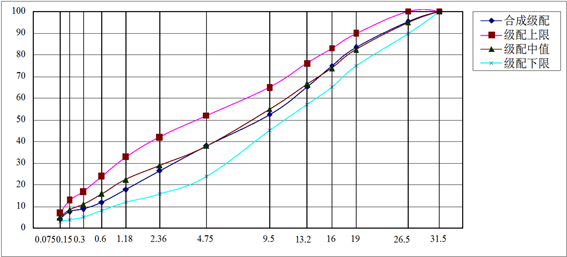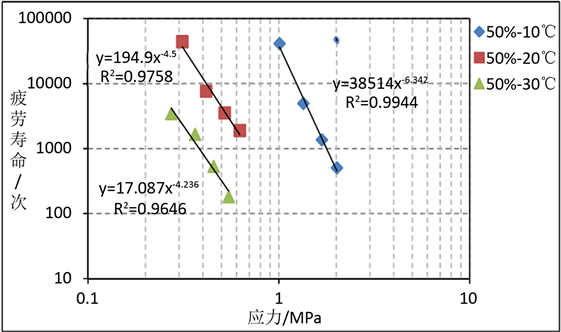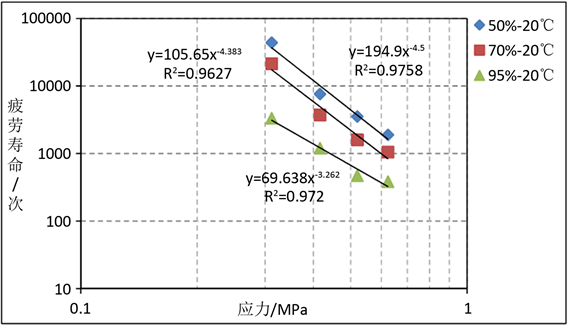﻿ Weibull分布模型在热再生沥青混合料疲劳特性分析中的应用研究

# Weibull分布模型在热再生沥青混合料疲劳特性分析中的应用研究Application of Weibull Distribution Model in Fatigue Analysis of Hot Recycled Asphalt Mixture

Abstract: In order to study the fatigue characteristic of hot recycled asphalt mixture, indirect tensile test was carried out aiming at hot recycled AC-25. In view of the randomness and discreteness of small sample fatigue test results, three-parameter Weibull distribution model was introduced to analyze test data. The results show that the fatigue life of hot recycled AC-25 obeys three-parameter Weibull distribution; the stress fatigue equation under different guarantee rates shows that the fatigue resistance of recycled asphalt mixture decreases greatly with the increase of temperature, but the sensitivity of fatigue performance to stress decreases at the same time; the appropriate guarantee rate should be selected to predict fatigue life of asphalt mixture according to the actual situation.

1. 引言

2. 热再生沥青混合料配合比设计

2.1. RAP

2.2. 热再生沥青混合料配合比Figure 1. Synthetic gradation curve of mixture

3. 间接拉伸疲劳试验

3.1. 试件制备

3.2. 试验参数

4. 试验结果及分析

4.1. 劈裂强度Table 2. Representative value of splitting strength

4.2. Weibull分布模型

$f\left(N\right)=\frac{b}{{N}_{a}-{N}_{0}}{\left(\frac{N-{N}_{0}}{{N}_{a}-{N}_{0}}\right)}^{b-1}\mathrm{exp}\left[-{\left(\frac{N-{N}_{0}}{{N}_{a}-{N}_{0}}\right)}^{b}\right]\text{\hspace{0.17em}}\text{\hspace{0.17em}}\text{\hspace{0.17em}}\left(N\ge {N}_{0}\right)$ (1)

N0为起始最小寿命参数；

Na为特征寿命参数；

b为形状参数。

$F\left(N\right)=p\left(n\le N\right)={\int }_{{N}_{0}}^{N}f\left(N\right)\text{d}N$ (2)

$F\left(N\right)=1-\mathrm{exp}\left[-{\left(\frac{N-{N}_{0}}{{N}_{a}-{N}_{0}}\right)}^{b}\right]$ (3)

$R\left(N\right)=\mathrm{exp}\left[-{\left(\frac{N-{N}_{0}}{{N}_{a}-{N}_{0}}\right)}^{b}\right]$ (4)

$\mathrm{ln}\left[\mathrm{ln}\frac{1}{R\left(N\right)}\right]=b\mathrm{ln}\left(N-{N}_{0}\right)-b\mathrm{ln}\left({N}_{a}-{N}_{0}\right)$ (5)

4.3. 疲劳寿命数据分析Table 3. Laboratory fatigue test resultsTable 4. Fitting result of fatigue life based on Weibull distributionTable 5. Fatigue life under different guaranteed rates

4.4. 疲劳寿命预估模型

$N=\kappa {\sigma }^{-n}$ (6)Figure 2. 50% guaranteed rate, stress fatigue equation under different temperaturesFigure 3. 20℃, stress fatigue equation under different guaranteed rates

5. 结论

1) 劈裂强度试验结果表明，热再生沥青混合料的劈裂强度随温度的升高而明显降低，这与普通沥青混合料的规律相一致，说明温度仍然是影响热再生沥青混合料性能的重要因素。

2) 间接拉伸疲劳试验结果表明，即使所有试验条件均相同，小样本疲劳试验结果仍然具有很大的离散性，采用正态分布或对数正态分布来分析热再生混合料疲劳特性存在很大的不准确性。

3) 热再生沥青混合料的疲劳试验结果服从三参数Weibull分布，可以拟合得到不同保证率下混合料的应力疲劳方程，疲劳方程显示，随着试验温度的升高，热再生沥青混合料的抗疲劳性能大幅度降低，同时混合料疲劳性能对应力的敏感程度也降低。

4) 在进行热再生沥青混合料疲劳寿命预估时，应根据实际情况综合考虑选取合适的保证率，保证率取值的大小决定了材料疲劳失效判定次数的高低。

 邹桂莲, 徐剑. 再生沥青混合料路用性能试验研究[J]. 公路与汽运, 2011(2): 121-124.

 熊巍, 卢何. 热再生沥青混合料的路用性能试验研究[J]. 公路, 2006(10): 191-194.

 何兆益, 陈龙, 陈先勇. 厂拌热再生沥青混合料力学性能及应用研究[J]. 建筑材料学报, 2016, 19(5): 871-875.

 张科, 郑鑫, 张旭. 热再生沥青混合料疲劳特性试验研究[J]. 公路工程, 2017, 42(1): 228-232.

 Huang, B., Zhang, Z. and Kinger. W. (2004) Fatigue Crack Characteristics of HMA Mixtures Containing RAP. Proceedings of 5th International RILEM Conference on Cracking in Pavements, Limoges, 2004, 631-638.

 兰青, 徐伟, 赵劲松, 等. 高比例RAP厂拌热再生沥青混合料在广惠高速公路试验与评价[J]. 公路工程, 2013, 38(4): 79-83.

 交通部公路科学研究所. JTG F40-2004公路沥青路面施工技术规范[S]. 北京: 人民交通出版社, 2004.

 交通部公路科学研究院. JTG E20-2011公路工程沥青及沥青混合料试验规程[S]. 北京: 人民交通出版社, 2011.

 黄琴龙, 权晨嘉, 杨壮, 等. 乳化沥青水泥稳定碎石混合料的疲劳性能试验[J]. 建筑材料学报, 2017, 20(5): 739-744.

 贾侃. 半刚性基层材料的疲劳特性研究[D]: [博士学位论文]. 西安: 长安大学, 2009.

Top